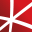# Top 20 NuGet fft Packages

Math.NET Numerics is the numerical foundation of the Math.NET project, aiming to provide methods and algorithms for numerical computations in science, engineering and every day use. Supports .Net Framework 4.0 or higher and .Net Standard 1.3 or higher, on Windows, Linux and Mac.## Math.NET Numerics - Strong Name Edition

Math.NET Numerics is the numerical foundation of the Math.NET project, aiming to provide methods and algorithms for numerical computations in science, engineering and every day use. Supports .Net Framework 4.0 or higher and .Net Standard 1.3 or higher, on Windows, Linux and Mac. This package contain...
F# Modules for Math.NET Numerics, the numerical foundation of the Math.NET project, aiming to provide methods and algorithms for numerical computations in science, engineering and every day use. Supports .Net Framework 4.5 or higher and .Net Standard 1.6 or higher, on Windows, Linux and Mac.## Math.NET Numerics for F# - Strong Name Edition

F# Modules for Math.NET Numerics, the numerical foundation of the Math.NET project, aiming to provide methods and algorithms for numerical computations in science, engineering and every day use. Supports .Net Framework 4.5 or higher and .Net Standard 1.6 or higher, on Windows, Linux and Mac. This pa...
A library for advanced numerical computing, with support for data wrangling, statistics, matrix algebra, advanced functions, numerical integration and optimization, and Fourier transforms.
Foundational classes for financial, engineering, and scientific applications, including complex number classes, general vector and matrix classes, structured sparse matrix classes and factorizations, general sparse matrix classes and factorizations, general matrix decompositions, least squares solut...
The GPU-accelerated version of package CenterSpace.NMath. With a few minor exceptions, such as optional GPU configuration settings, the API is identical between CenterSpace.NMath.Premium and CenterSpace.NMath. If using at least .NET Framework 4.6.1 or .NET Core 2.0, we recommend using one of our NMa...## NMath - Standard Library - Windows x64

Foundational classes for financial, engineering, and scientific applications, including complex number classes, general vector and matrix classes, structured sparse matrix classes and factorizations, general sparse matrix classes and factorizations, general matrix decompositions, least squares solut...
Foundational classes for financial, engineering, and scientific applications, including complex number classes, general vector and matrix classes, structured sparse matrix classes and factorizations, general sparse matrix classes and factorizations, general matrix decompositions, least squares solut...
The Extreme Optimization Numerical Libraries for .NET are a set of libraries for numerical computing and data analysis. This is the main package that contains all the core functionality. For optimal performance, we strongly recommend also referencing one of the native packages based on Intel's Mat...
Intel® Math Kernel Library (Intel® MKL) is a computing math library of highly optimized, extensively threaded routines for applications that require maximum performance. This package provides C programming language interfaces. Intel MKL C language interfaces can be called from applications written i...
Intel® Math Kernel Library (Intel® MKL) is a computing math library of highly optimized, extensively threaded routines for applications that require maximum performance. This package provides C programming language interfaces. Intel MKL C language interfaces can be called from applications written i...
Intel® Math Kernel Library (Intel® MKL) is a computing math library of highly optimized, extensively threaded routines for applications that require maximum performance. This package provides C programming language interfaces. Intel MKL C language interfaces can be called from applications written i...
Extreme Optimization Numerical Libraries for .NET Data Access Library for JSON.## NMath - Standard Library - OSX x64 (alpha)

Foundational classes for financial, engineering, and scientific applications, including complex number classes, general vector and matrix classes, structured sparse matrix classes and factorizations, general sparse matrix classes and factorizations, general matrix decompositions, least squares solut...
Intel® Math Kernel Library (Intel® MKL) is a computing math library of highly optimized, extensively threaded routines for applications that require maximum performance. This package provides C programming language interfaces. Intel MKL C language interfaces can be called from applications written i...
Intel® Math Kernel Library (Intel® MKL) is a computing math library of highly optimized, extensively threaded routines for applications that require maximum performance. This package provides C programming language interfaces. Intel MKL C language interfaces can be called from applications written i...
Intel® Math Kernel Library (Intel® MKL) is a computing math library of highly optimized, extensively threaded routines for applications that require maximum performance. This package provides C programming language interfaces. Intel MKL C language interfaces can be called from applications written i...
Intel® Math Kernel Library (Intel® MKL) is a computing math library of highly optimized, extensively threaded routines for applications that require maximum performance. This package provides C programming language interfaces. Intel MKL C language interfaces can be called from applications written i...
Intel® Math Kernel Library (Intel® MKL) is a computing math library of highly optimized, extensively threaded routines for applications that require maximum performance. This package provides C programming language interfaces. Intel MKL C language interfaces can be called from applications written i...